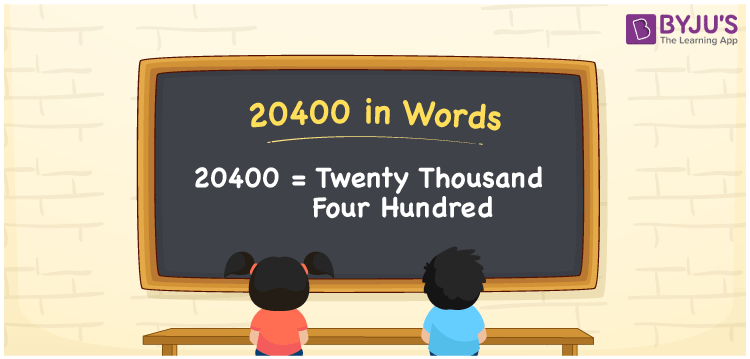# 20400 in Words

20400 in words is Twenty Thousand Four Hundred. For example, if you spend Rs. 20400 in a month, you can write, “I have spent Rs. Twenty Thousand Four Hundred in a month”. In general, the number name of any number can be written using the ones, tens, hundreds, and thousands place of a number. Thus, the place value chart is useful for writing the number 20400 in words.

 20400 in words Twenty Thousand Four Hundred Twenty Thousand Four Hundred in Numbers 20400

## 20400 in English Words## How to Write 20400 in Words?

We can convert 20400 to words using a place value chart. This can be done as follows. The number 20400 has 5 digits, so let’s make a chart that shows the place value up to 5 digits.

 Ten thousands Thousands Hundreds Tens Ones 2 0 4 0 0

Thus, we can write the expanded form as:

2 × Ten thousand + 0 × Thousand + 4 × Hundred + 0 × Ten + 0 × One

= 2 × 10000 + 0 × 1000 + 4 × 100 + 0 × 10 + 0 × 1

= 20400

= Twenty Thousand Four Hundred

20400 is the natural number that is succeeded by 20399 and preceded by 20401.

20400 in words – Twenty Thousand Four Hundred

Is 20400 an odd number? – No.

Is 20400 an even number? – Yes

Is 20400 a perfect square number? – No

Is 20400 a perfect cube number? – No

Is 20400 a prime number? – No

Is 20400 a composite number? – Yes

## Frequently Asked Questions on 20400 in Words

Q1

### How to write 20400 in words?

20400 in English is written as “Twenty Thousand Four Hundred”.
Q2

### Is the number 20400 an odd number?

No, the number 20400 is not an odd number.
Q3

### Is 20400 a composite number?

Yes, 20400 is a composite number.